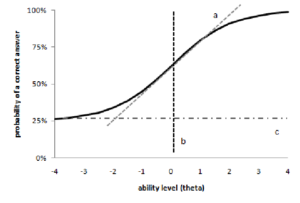Item Response Theory

Item response theory (IRT) is also known as modern testing theory or latent trait theory.  In classical test theory, items on a scale measuring a single construct are generally considered to be equivalent to each other.  IRT measures the specific characteristics of each item.  An item characteristic curve (ICC) can be defined for each item.  In this way, equivalent scales can be constructed by choosing items shown to be equivalent.  One can also pick items that best measure a particular ability level and one can measure whether items function equivalently when applied to different populations or when translated into different languages.

The ICC is defined with a curve that has from one to three parameters.  These parameters define a function that increases as theta (q) increases.  Theta is the strength of the latent trait or ability level of a particular individual. On an IQ test, higher theta would represent higher intelligence.  On a measure of anxiety, higher theta would reflect higher anxiety levels.

The three parameters are:

a: Discrimination or slope.  Classical test theory assumes that the test is equally discriminating at all levels of ability and is shown as the correlation between an item and the total test score.  IRT shows that an item has optimal discrimination at a particular ability level.

b: Item difficulty.  As b increases, the probability of passing or endorsing the item goes down.

c: Adjustment for guessing:  On a multiple choice test with four options, someone with very low theta (no ability on the latent trait) would theoretically score 25%.  This is the left asymptote of the ICC, where the curve levels off as it goes to the left.

A typical ICC is shown below.  The x-axis (horizontal) reflects theta, the ability level (or latent trait level) of the test taker.  The y-axis (vertical) reflects the probability of passing or endorsing an item.  At high levels of the latent trait, the chance of passing the item is almost certain.  At low levels, the chance of passing the item falls to “c” or the chance of guessing correctly.